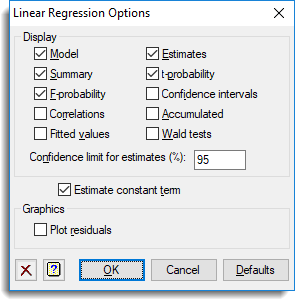1. Home
2. Linear Regression Options

# Linear Regression Options

Select the output to be generated initially in a regression analysis – the same information can also be displayed after the analysis, using the Further output dialog. You can also request that no constant term is included in the model: this will affect only the parameterization of factor effects, if there are factors in the model; but if not, it will constrain the regression to pass through the origin.

For a general regression model, you can also control the maximum order of interaction to be generated when you use model-formula operators like *. The default is to include all interactions, up to those involving nine variates or factors. (You cannot ask for more than nine.)## Display

 Model Details of the model that is fitted. Summary Summary analysis-of-variance. F-probability F probabilities for variance ratios. Correlations Correlations between the parameter estimates Fitted values Table containing the values of the response variate, the fitted values and residuals from the regression. Estimates Estimates of the parameters in the model t-probability t probabilities for the parameter estimates the fitted values, standardized residuals and leverages. Confidence intervals Confidence intervals for the parameter estimates. The confidence limit can specified as a percentage using the Confidence limit for estimates (%) field. Accumulated Analysis of variance table containing a line for each change in the fitted model. Wald tests Wald and F tests for dropping terms from a regression. Where the regression contains a model function (e.g. polynomial, smoothing spline or locally weighted (loess) terms), the Wald tests cannot be calculated.

## Estimate constant term

Specifies whether to include a constant in the regression model. In simple linear regression this omits the intercept, in other words the fitted line is constrained to pass through the origin.

## Graphics

Lets you generate default plots when you run a regression analysis. Plot residuals will produce diagnostic plots of the residuals. Following simple linear regression you can also use Plot fitted model to generate a graph of the fitted model.